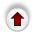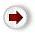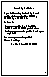In open addressing, each position in the array is in one of three states, EMPTY, DELETED, or OCCUPIED. If a position is OCCUPIED, it contains a legitimate value (key and data); otherwise, it contains no value. Positions are initially EMPTY. If the value in a position is deleted, the position is marked as DELETED, not EMPTY: the need for this distinction is explained below.

The algorithm for inserting a value V into a table is this:

1. compute the position at which V ought to be stored, P = H(key(V)).
2. If position P is not OCCUPIED, store V there.
3. If position P is OCCUPIED, compute another position in the table; set P to this value and repeat (2)-(3).
How should we ``compute another position'' in step (3)?

### Linear Probing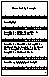The simplest method is called linear probing. P = (1 + P) mod TABLE_SIZE. This has the virtues that it is very fast to compute and that it `probes' (i.e. looks at) every position in the hash table. To see its fatal drawback, consider the following example.

In this example, the table is size 10, keys are 2-digit integers, and H(k) = k mod 10. Initially all the positions in the table are EMPTY:

`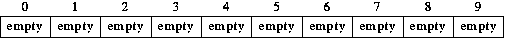`

If we now insert 15, 17, and 8, there are no collisions; the result is:

`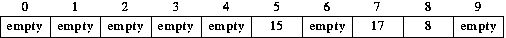`

When we insert 35, we compute P = H(35) = 35 mod 10 = 5. Position 5 is occupied (by 15). So we must look elsewhere for a position in which to store 35. Using linear probing, the position we would try next is 5+1 mod 10 = 6. Position 6 is empty, so 35 is inserted there.

`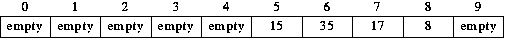`

Now suppose we insert 25. We first try position (25 mod 10). It is occupied. Using linear probing we next try position 6 (5+1 mod 10). It too is occupied. Next we try position 7 (6+1 mod 10); it is occupied, as is the next one we try, position 8 (7+1 mod 10). Position 9 is next (8+1 mod 10); it is empty so 25 is inserted there.

`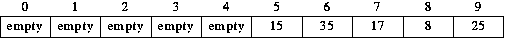`

Now suppose we insert 75. The first position we try is 5. Then we will try position 6, then 7, then 8, then 9. Finally we try position 0 (9+1 mod 10); it is empty so 75 is stored there.

`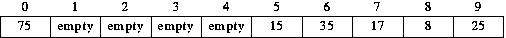`

Has anyone spotted the flaw in the linear probing technique? Think about this: what would happen if we now inserted 85, then 95, then 55?

Answer: Each one would probe exactly the same positions as its predecessors. This phenomenon is known as clustering. It leads to very inefficient operations, because it causes the number of collisions to be much greater than it need be. To eliminate clustering each different key should probe the table in a different order.

### Double Hashing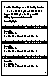A very simple way to sharply reduce clustering is to increment P, not by a constant (as is done in linear probing) but, by an amount that depends on the Key. We thus have a second hashing function, INCREMENT(Key). Then instead of

P = (1 + P) mod TABLE_SIZE

we use

P = (P + INCREMENT(Key)) mod TABLE_SIZE

This technique is called double hashing.

In our example, suppose INCREMENT(Key) = 1 + (Key mod 7) - we add 1 to ensure that our increment is never 0! - 15, 17, and 8, would be inserted as before, since there were no collisions:

``

When we insert 35, we compute P = H(35) = 35 mod 10 = 5. Position 5 is occupied (by 15). So we must look elsewhere for a position in which to store 35. Using double hashing, the next position we consider is determined by the INCREMENT function. It so happens that INCREMENT(35) equals 1, so 35 would be treated just the same with double hashing as with linear probing. Adding this increment to the current position leads us to probe position 6; it is empty, so 35 is inserted there.

``

Now suppose we insert 25. With linear probing, this followed the same probing sequence as 15 and 35, but with double hashing it will not. It is initially hashed to position 5, which is occupied. The next position we try is determined by the INCREMENT function. INCREMENT(35) was 1, but INCREMENT(25) is 5, so the next position we look at it is (5+5 mod 10), i.e. position 0. This position is not occupied, so 25 is stored there.

`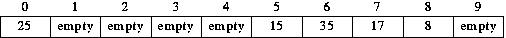`

Now we insert 75. It is initially mapped to position 5, which is occupied. INCREMENT(75) = 6, so the next position we probe is (5+6 mod 10), position 1. It is not occupied, so 75 is stored there.

As you can see, each value probes the array positions in a different order. There is no clustering: if two keys probe the same position, the next position they probe is different. Of course, there do exist keys that have the same value of H(Key) and the same value of INCREMENT(Key), but these are much rarer than keys that just have the same H(Key) value.

With any open addressing technique, it is necessary to distinguish between EMPTY positions - which have never been occupied - and positions which once were occupied but now are not (because the value stored there has been deleted). The INSERT operation ignores this distinction, but the RETRIEVE and DELETE operations treat the two kinds of ``unoccupied'' positions very differently; in fact, they treat DELETED positions exactly the same as OCCUPIED ones (whereas INSERT treats DELETED positions exactly the same as EMPTY ones).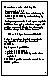The algorithm for retrieving a value in the table with key K is this:

1. P = H(K), the position where the key would be found if no collisions occurred when it was inserted.

2. If position P is EMPTY, then no value with key K occurs in the table

3. If position P is OCCUPIED by a value whose key K, return the value in position P

4. Otherwise, compute another position in the table; set P to this value and repeat (2)-(4).

This algorithm needs one additional stopping criterion. In some circumstances - for instance when all the positions are marked as DELETED - the stopping conditions in steps (2) and (3) will never be true; as written, the algorithm will never stop. This can be corrected by testing if P == H(K), i.e. if we have come full circle in our probing sequence.

The DELETE operation is identical, except that in step (3) position P would simply be marked as DELETED.

The ``otherwise'' in step (4) captures two distinct possibilities: that the position is marked DELETED, or that it is OCCUPIED by a value whose key is not K. Why should DELETED be treated in this way? Why can't it be treated like EMPTY ?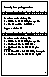To see why DELETED must be treated like OCCUPIED, not EMPTY, let us return to the example hash table created using using linear probing. The same explanation applies to any form of open addressing but it is most easily illustrated with linear probing. The result of several insertions using linear probing, was:

``

Suppose we are asked to retrieve the value with key 80.

80 is hashed to position 0, which is OCCUPIED with a value whose key is not 80. Using linear probing, we'd next look at position 1 (0+1 mod 10). This position is EMPTY. We can immediately be sure that 80 is not in the table. If it were in the table, position 1 could not be empty, because 80 would have been put there by the INSERT algorithm. The point is that all the operations follow the same probing sequence - they must do so if RETRIEVE and DELETE are to have any hope of finding the values inserted by INSERT.

Suppose now we DELETE 35. It is hashed to position 5, which is occupied, and, using linear probing, we look next in position 6, where we find what we are looking for. Position 6 is marked as DELETED, not EMPTY.

`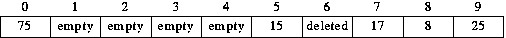`

To see the difference, consider now what will happen if we try to retrieve 25. When 25 was inserted, its probing sequence took it to position 6. The same will happen now, during retrieval. When 25 was inserted, position 6 was occupied by 35, so 25's probing sequence continued past position 6, ultimately ending at position 9. We must arrange for the same probing sequence to occur now, even though some of the positions in the original probing sequence are no longer occupied. That is why we must distinguish between DELETED positions and EMPTY ones. In our search for 25 we must not stop at position 6; therefore we must not mark it as EMPTY.

When we are searching for a value that is not in the table, the presence of positions marked DELETED is a source of great inefficiency. In the worst case, all positions are marked DELETED: to determine that a value is not in the table we must look at every position in the table.